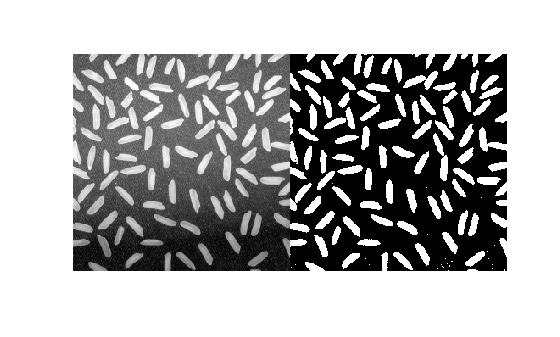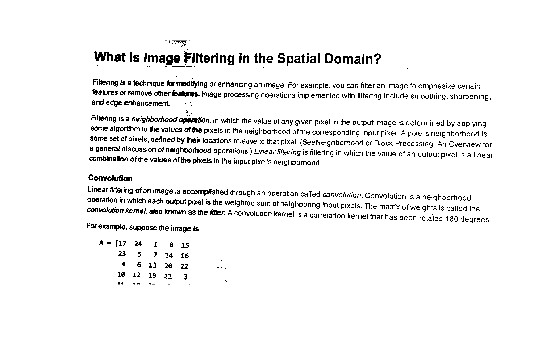Adaptive image threshold using local first-order statistics

## Syntax

``T = adaptthresh(I)``
``T = adaptthresh(I,sensitivity)``
``T = adaptthresh(___,Name,Value)``

## Description

example

````T = adaptthresh(I)` computes a locally adaptive threshold for 2-D grayscale image or 3-D grayscale volume `I`. The `adaptthresh` function chooses the threshold based on the local mean intensity (first-order statistics) in the neighborhood of each pixel. The threshold `T` can be used with the `imbinarize` function to convert the grayscale image to a binary image.```

example

````T = adaptthresh(I,sensitivity)` computes a locally adaptive threshold with sensitivity factor specified by `sensitivity`. `sensitivity` is a scalar in the range [0,1] that indicates sensitivity towards thresholding more pixels as foreground.```

example

````T = adaptthresh(___,Name,Value)` computes a locally adaptive threshold using name-value pairs to control aspects of the thresholding.```

## Examples

collapse all

`I = imread('rice.png');`

Use `adaptthresh` to determine threshold to use in binarization operation.

`T = adaptthresh(I, 0.4);`

Convert image to binary image, specifying the threshold value.

`BW = imbinarize(I,T);`

Display the original image with the binary version, side-by-side.

```figure imshowpair(I, BW, 'montage')````I = imread('printedtext.png');`

Using `adaptthresh` compute adaptive threshold and display the local threshold image. This represents an estimate of average background illumination.

```T = adaptthresh(I,0.4,'ForegroundPolarity','dark'); figure imshow(T)```Binarize image using locally adaptive threshold

```BW = imbinarize(I,T); figure imshow(BW)```Load 3-D volume into the workspace.

```load mristack; V = mristack;```

Display the data.

```figure slice(double(V),size(V,2)/2,size(V,1)/2,size(V,3)/2) colormap gray shading interp```Calculate the threshold.

`J = adaptthresh(V,'neigh',[3 3 3],'Fore','bright');`

Display the threshold.

```figure slice(double(J),size(J,2)/2,size(J,1)/2,size(J,3)/2) colormap gray shading interp```## Input Arguments

collapse all

Grayscale image or volume, specified as a 2-D numeric matrix or 3-D numeric array.

If the image contains `Inf`s or `NaN`s, the behavior of `adaptthresh` is undefined. Propagation of `Inf`s or `NaN`s might not be localized to the neighborhood around `Inf` or `NaN` pixels.

Data Types: `single` | `double` | `int8` | `int16` | `int32` | `uint8` | `uint16` | `uint32`

Determine which pixels get thresholded as foreground pixels, specified as a number in the range [0, 1]. High sensitivity values lead to thresholding more pixels as foreground, at the risk of including some background pixels.

Data Types: `single` | `double` | `int8` | `int16` | `int32` | `int64` | `uint8` | `uint16` | `uint32` | `uint64`

### Name-Value Arguments

Specify optional pairs of arguments as `Name1=Value1,...,NameN=ValueN`, where `Name` is the argument name and `Value` is the corresponding value. Name-value arguments must appear after other arguments, but the order of the pairs does not matter.

Before R2021a, use commas to separate each name and value, and enclose `Name` in quotes.

Example: `T = adaptthresh(I,0.4,'ForegroundPolarity','dark');`

Size of neighborhood used to compute local statistic around each pixel, specified as a positive odd integer or a 2-element vector of positive odd integers.

Data Types: `single` | `double` | `int8` | `int16` | `int32` | `int64` | `uint8` | `uint16` | `uint32` | `uint64`

Determine which pixels are considered foreground pixels, specified using one of the following:

Value

Meaning

`'bright'`

The foreground is brighter than the background.

`'dark'`

The foreground is darker than the background

Data Types: `char` | `string`

Statistic used to compute local threshold at each pixel, specified as one of the following:

Value

Meaning

`'mean'`

The local mean intensity in the neighborhood. This technique is also called Bradley’s method .

`'median'`

The local median in the neighborhood. Computation of this statistic can be slow. Consider using a smaller neighborhood size to obtain faster results.

`'gaussian'`

The Gaussian weighted mean in the neighborhood.

Data Types: `char` | `string`

## Output Arguments

collapse all

Normalized intensity values, returned as a numeric matrix or numeric array of the same size as the input image or volume, `I`. Values are normalized to the range [0, 1].

Data Types: `double`

 Bradley, D., G. Roth, "Adapting Thresholding Using the Integral Image," Journal of Graphics Tools. Vol. 12, No. 2, 2007, pp.13–21.